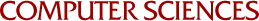## Characterization of Linear Complementarity Problems as Linear Programs

Olvi Mangasarian
1976

It is shown that the linear complementarity problem of finding an n-by-1 vector x such that Mx + q > 0, x > 0, and xT(Mx+q) = 0, where M is a given n-by-n real matrix and q is a given n-by-l vector, is solvable if and only if the linear program: minimize pTx subject to Mx + q > 0, x > 0, is solvable, where p is an n-by-1 vector which satisfies certain conditions. Furthermore each so1ution of the linear program, solves the linear complementarity problem. For a number of special cases including those when M has nonpositive off-diagonal elements, or when M is strictly or irreducib1y diagonally dominant, or when M is a positive matrix with a dominant diagonal columnwise, p is very easily determined and the linear complementarity problem can be solved as an ordinary 1inear program. Examples of linear complementarity problems are given which can be solved as linear programs, but not by Lemke's method or the principal pivoting method.